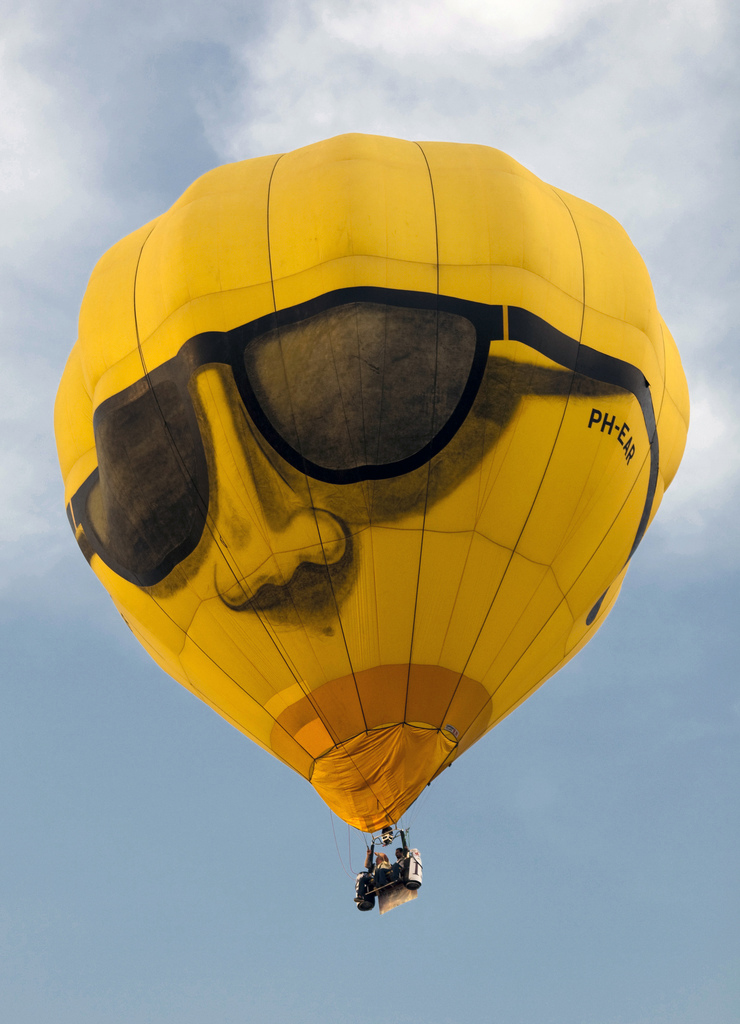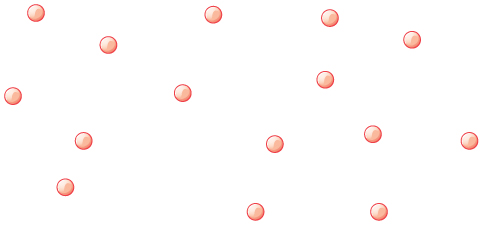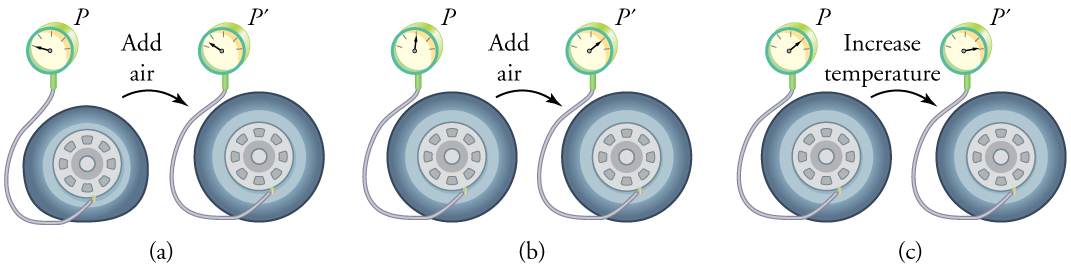# 13.3 The ideal gas law

 Page 1 / 11
• State the ideal gas law in terms of molecules and in terms of moles.
• Use the ideal gas law to calculate pressure change, temperature change, volume change, or the number of molecules or moles in a given volume.
• Use Avogadro’s number to convert between number of molecules and number of moles.The air inside this hot air balloon flying over Putrajaya, Malaysia, is hotter than the ambient air. As a result, the balloon experiences a buoyant force pushing it upward. (credit: Kevin Poh, Flickr)

In this section, we continue to explore the thermal behavior of gases. In particular, we examine the characteristics of atoms and molecules that compose gases. (Most gases, for example nitrogen, ${\text{N}}_{2}$ , and oxygen, ${\text{O}}_{2}$ , are composed of two or more atoms. We will primarily use the term “molecule” in discussing a gas because the term can also be applied to monatomic gases, such as helium.)

Gases are easily compressed. We can see evidence of this in [link] , where you will note that gases have the largest coefficients of volume expansion. The large coefficients mean that gases expand and contract very rapidly with temperature changes. In addition, you will note that most gases expand at the same rate, or have the same $\beta$ . This raises the question as to why gases should all act in nearly the same way, when liquids and solids have widely varying expansion rates.

The answer lies in the large separation of atoms and molecules in gases, compared to their sizes, as illustrated in [link] . Because atoms and molecules have large separations, forces between them can be ignored, except when they collide with each other during collisions. The motion of atoms and molecules (at temperatures well above the boiling temperature) is fast, such that the gas occupies all of the accessible volume and the expansion of gases is rapid. In contrast, in liquids and solids, atoms and molecules are closer together and are quite sensitive to the forces between them.Atoms and molecules in a gas are typically widely separated, as shown. Because the forces between them are quite weak at these distances, the properties of a gas depend more on the number of atoms per unit volume and on temperature than on the type of atom.

To get some idea of how pressure, temperature, and volume of a gas are related to one another, consider what happens when you pump air into an initially deflated tire. The tire’s volume first increases in direct proportion to the amount of air injected, without much increase in the tire pressure. Once the tire has expanded to nearly its full size, the walls limit volume expansion. If we continue to pump air into it, the pressure increases. The pressure will further increase when the car is driven and the tires move. Most manufacturers specify optimal tire pressure for cold tires. (See [link] .)(a) When air is pumped into a deflated tire, its volume first increases without much increase in pressure. (b) When the tire is filled to a certain point, the tire walls resist further expansion and the pressure increases with more air. (c) Once the tire is inflated, its pressure increases with temperature.

#### Questions & Answers

How do you convert 0.0045kgcmÂ³ to the si unit?
how many state of matter do we really have like I mean... is there any newly discovered state of matter?
I only know 5: •Solids •Liquids •Gases •Plasma •Bose-Einstein condensate
Thapelo
Alright Thank you
Falana
Which one is the Bose-Einstein
James
can you explain what plasma and the I her one you mentioned
Olatunde
u can say sun or stars are just the state of plasma
Mohit
but the are more than seven
Issa
what the meaning of continuum
What state of matter is fire
fire is not in any state of matter...fire is rather a form of energy produced from an oxidising reaction.
Xenda
Isn`t fire the plasma state of matter?
Walter
How can you define time?
Time can be defined as a continuous , dynamic , irreversible , unpredictable quantity .
Tanaya
what is the relativity of physics
How do you convert 0.0045kgcm³ to the si unit?
flint
What is the formula for motion
V=u+at V²=u²-2as
flint
S=ut+½at
flint
they are eqns of linear motion
King
S=Vt
Thapelo
v=u+at s=ut+at^\2 v^=u^+2as where ^=2
King
hi
hello
King
Explain dopplers effect
Not yet learnt
Bob
Explain motion with types
Bob
Acceleration is the change in velocity over time. Given this information, is acceleration a vector or a scalar quantity? Explain.
Scalar quantity Because acceleration has only magnitude
Bob
acleration is vectr quatity it is found in a spefied direction and it is product of displcemnt
bhat
its a scalar quantity
Paul
velocity is speed and direction. since velocity is a part of acceleration that makes acceleration a vector quantity. an example of this is centripetal acceleration. when you're moving in a circular patter at a constant speed, you are still accelerating because your direction is constantly changing.
Josh
acceleration is a vector quantity. As explained by Josh Thompson, even in circular motion, bodies undergoing circular motion only accelerate because on the constantly changing direction of their constant speed. also retardation and acceleration are differentiated by virtue of their direction in
fitzgerald
respect to prevailing force
fitzgerald
What is the difference between impulse and momentum?
Manyo
Momentum is the product of the mass of a body and the change in velocity of its motion. ie P=m(v-u)/t (SI unit is kgm/s). it is literally the impact of collision from a moving body. While Impulse is the product of momentum and time. I = Pt (SI unit is kgm) or it is literally the change in momentum
fitzgerald
Or I = m(v-u)
fitzgerald
Calculation of kinetic and potential energy
K.e=mv² P.e=mgh
Malia
K is actually 1/2 mv^2
Josh
what impulse is given to an a-particle of mass 6.7*10^-27 kg if it is ejected from a stationary nucleus at a speed of 3.2*10^-6ms²? what average force is needed if it is ejected in approximately 10^-8 s?
John
speed=velocity÷time velocity=speed×time=3.2×10^-6×10^-8=32×10^-14m/s impulse [I]=∆momentum[P]=mass×velocity=6.7×10^-27×32×10^-14=214.4×10^-41kg/ms force=impulse÷time=214.4×10^-41÷10^-8=214.4×10^-33N. dats how I solved it.if wrong pls correct me.
Melody
what is sound wave
sound wave is a mechanical longitudinal wave that transfers energy from one point to another
Ogor
its a longitudnal wave which is associted wth compresion nad rearfractions
bhat
what is power
it's also a capability to do something or act in a particular way.
Kayode
Newton laws of motion
Mike
power also known as the rate of ability to do work
Slim
power means capabilty to do work p=w/t its unit is watt or j/s it also represents how much work is done fr evry second
bhat
what does fluorine do?
strengthen and whiten teeth.
Gia
a simple pendulum make 50 oscillation in 1minute, what is it period of oscillation?
length of pendulm?
bhat
what is the difference between temperature and heat transfer?
temperature is the measurement of hotness or coldness of a body... heat transfer is the movement of heat from one body to another
Doc
U get it right
Titilayo
correct
PROMISE
heat is an energy possesed by any substance due to random kinetic energy possesed by molecules while temperature is driving force which gives direction of flow heat
bhatByBy Mistry BhaveshBy